• 欢迎大家分享资料！前往留言板评论即可！

# 16-GPRS(Air202)关于多个文件中的变量调用和定时器

1年前 (2021-05-15) 251次浏览

https://www.cnblogs.com/yangfengwu/p/9968405.html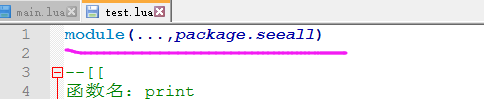实际上，这是lua5.几新加的功能，就是为了省掉那些复杂的程序

我又新建了一个test1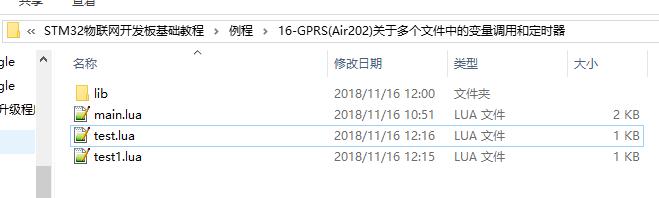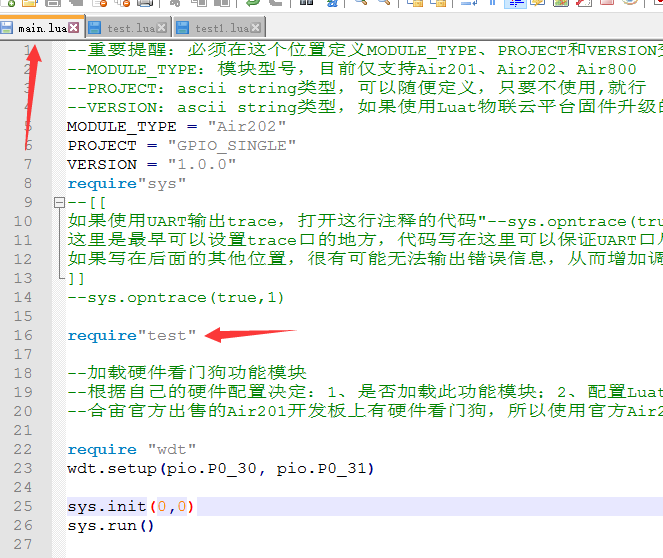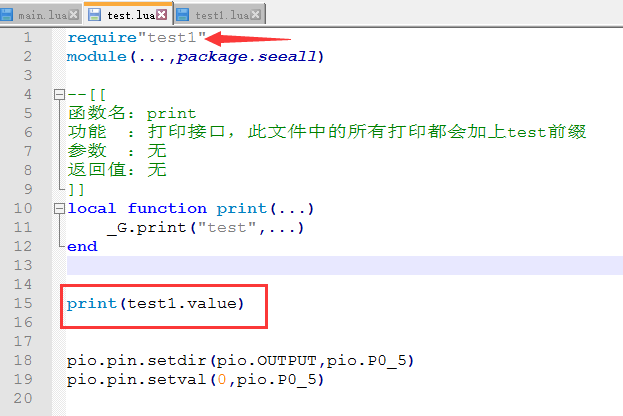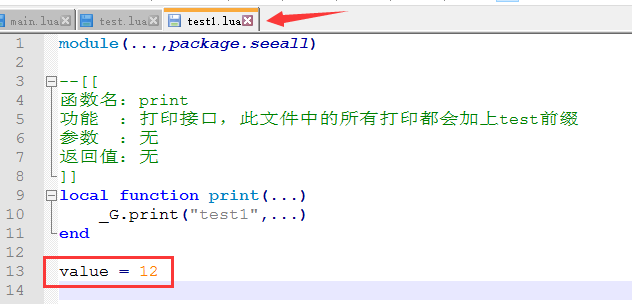下载进去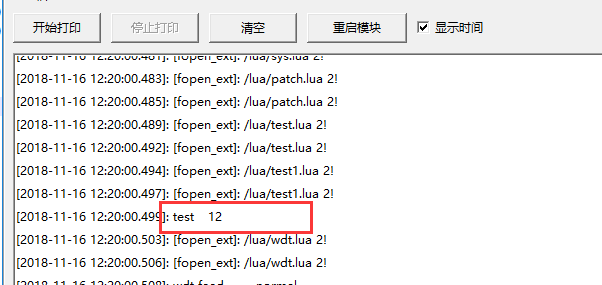注意哈，这样是不允许的，再用test1请求test文件，然后调用test里面的值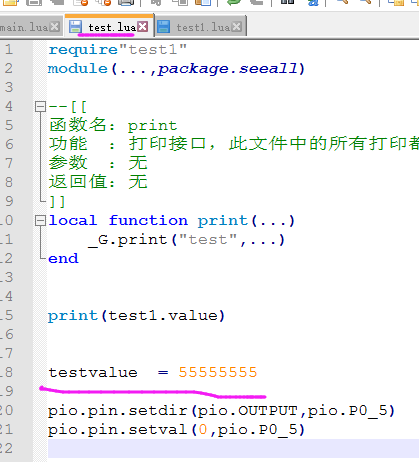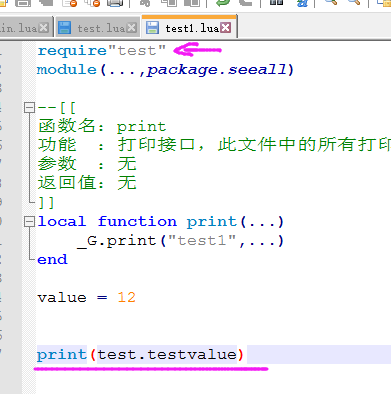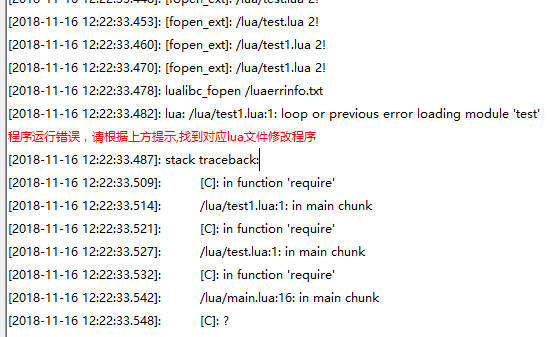如果想这样做，其实可以再做一个文件哈，里面放一个变量，然后test和test1都可以请求这个文件并操作这个变量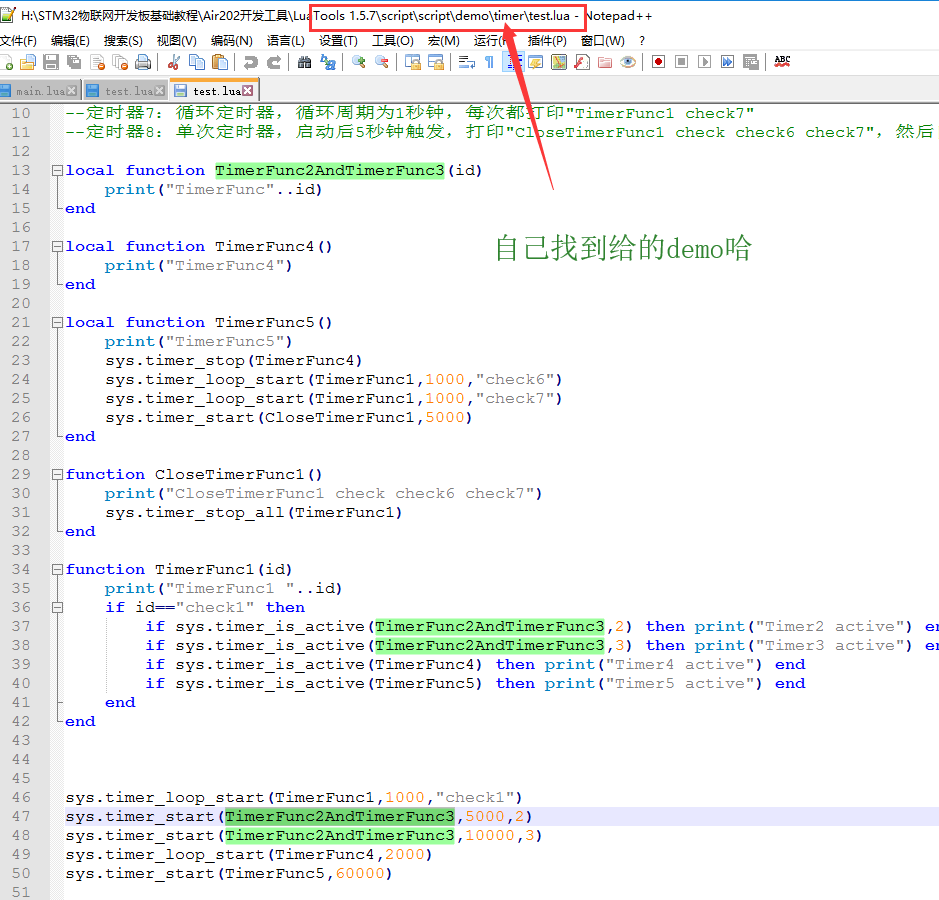module(...,package.seeall)

--[[

]]
local function print(...)
_G.print("test",...)
end

local function Timer1()
print("一次的定时器，没有ID")
end

sys.timer_start(Timer1,10000)//延时10S调用Timer1函数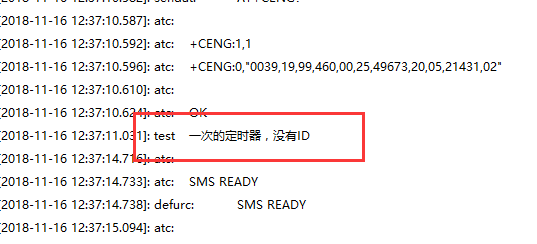一次性的不需要关闭，因为是自己关闭

module(...,package.seeall)

--[[

]]
local function print(...)
_G.print("test",...)
end

local function Timer1()
print("一次的定时器，没有ID")
end

sys.timer_start(Timer1,10000)

local function Timer2()
print("这个是循环定时器，没有ID")
end

sys.timer_loop_start(Timer2,2000)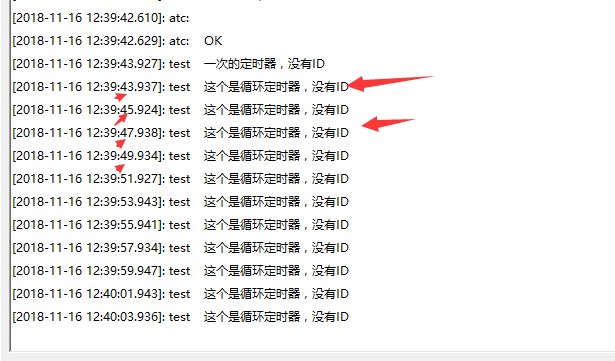现在用一个变量在里面加一，加到5停止这个定时器

module(...,package.seeall)

--[[

]]
local function print(...)
_G.print("test",...)
end

local function Timer1()
print("一次的定时器，没有ID")
end

sys.timer_start(Timer1,10000)

local cnt=0
local function Timer2()
print("这个是循环定时器，没有ID")    cnt=cnt+1
if  cnt>=5 then
cnt=0
sys.timer_stop(Timer2)--直接填入函数停止        print("这个是循环定时器，没有ID，现在停止")
end
end

sys.timer_loop_start(Timer2,2000)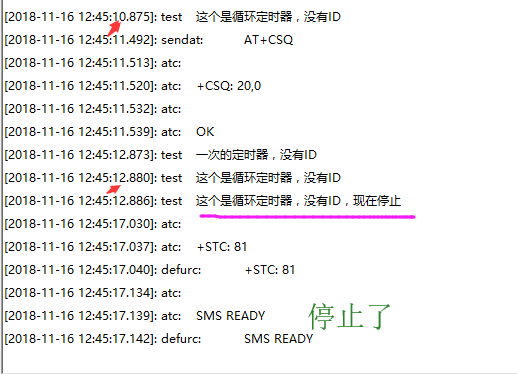现在说带ID的，可以看一下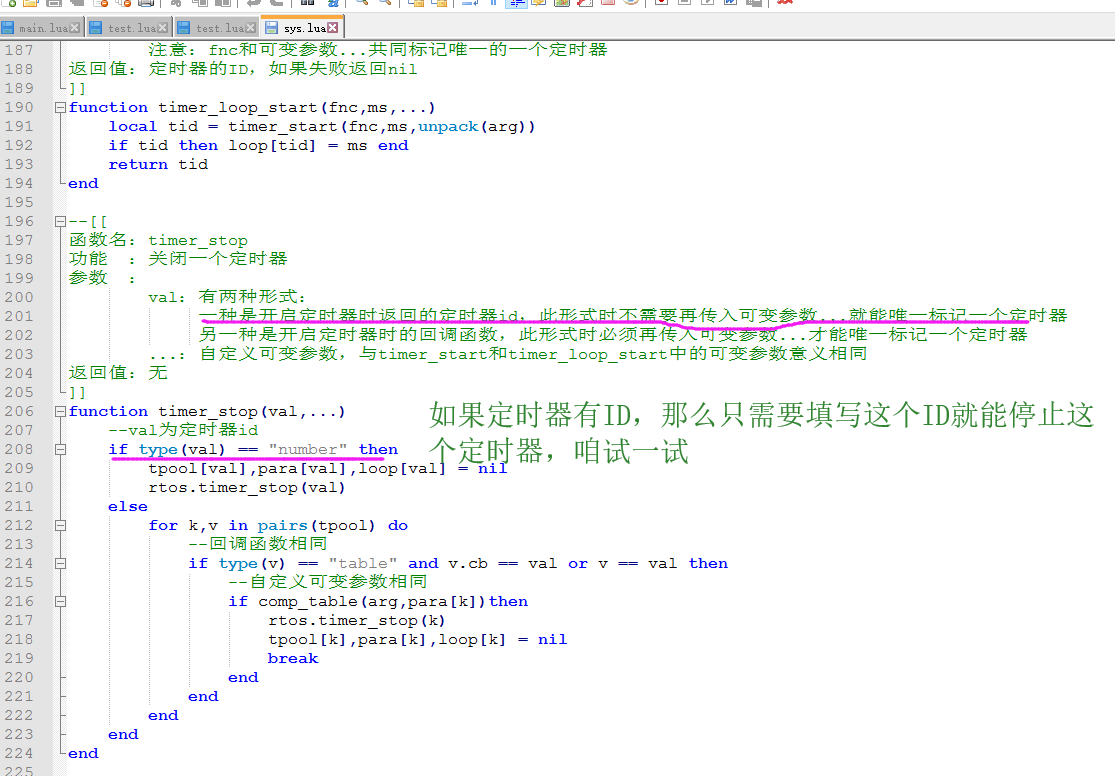module(...,package.seeall)

--[[

]]
local function print(...)
_G.print("test",...)
end

local function Timer1()
print("一次的定时器，没有ID")
end

sys.timer_start(Timer1,10000)

local cnt=0
local function Timer2()
print("这个是循环定时器，没有ID")
cnt=cnt+1
if  cnt>=5 then
cnt=0
sys.timer_stop(Timer2)--直接填入函数停止
print("这个是循环定时器，没有ID，现在停止")
end
end

sys.timer_loop_start(Timer2,2000)

local cnt1=0
local function Timer3()
print("这个是循环定时器，有ID")
cnt1=cnt1+1
if  cnt1>=5 then
cnt1=0
sys.timer_stop(1)--直接填入函数停止
print("这个是循环定时器，有ID，现在停止")
end
end

sys.timer_loop_start(Timer3,2000,1)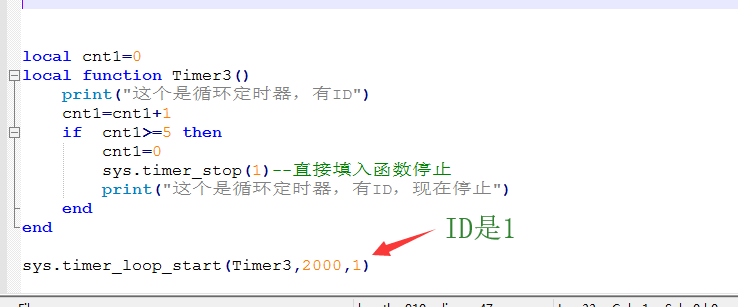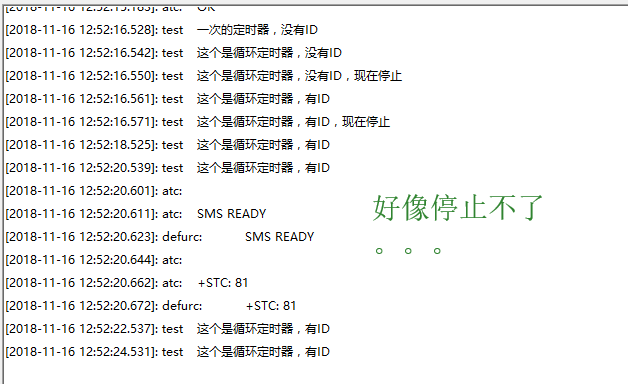所以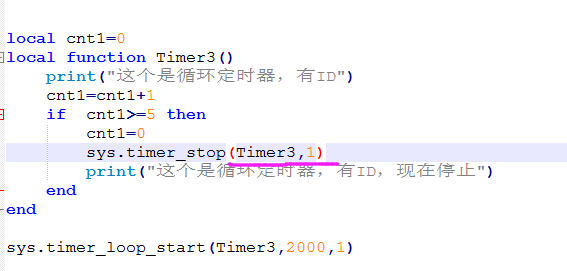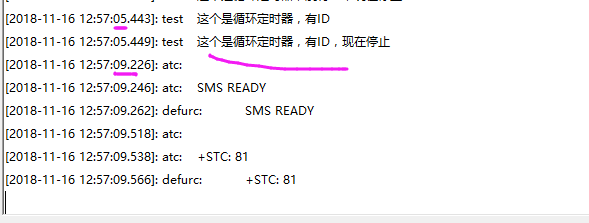这样可以停止

好现在控制灯每进一次定时器就反转一次，就是周期性亮灭

local function Timer4()
pio.pin.setval(1-pio.pin.getval(pio.P0_5),pio.P0_5)
end
sys.timer_loop_start(Timer4,2000,1)

1-pio.pin.getval(pio.P0_5)
pio.pin.getval(pio.P0_5)是读取引脚的高低电平状态   1-这个（0或者1） 正好是反转得到1和0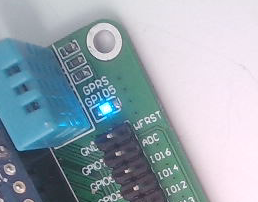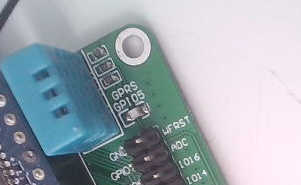最终所有的源码

module(...,package.seeall)

--[[

]]
local function print(...)
_G.print("test",...)
end

local function Timer1()
print("一次的定时器，没有ID")
end
sys.timer_start(Timer1,10000)

local cnt=0
local function Timer2()
print("这个是循环定时器，没有ID")
cnt=cnt+1
if  cnt>=5 then
cnt=0
sys.timer_stop(Timer2)--直接填入函数停止
print("这个是循环定时器，没有ID，现在停止")
end
end
sys.timer_loop_start(Timer2,2000)

local cnt1=0
local function Timer3()
print("这个是循环定时器，有ID")
cnt1=cnt1+1
if  cnt1>=5 then
cnt1=0
sys.timer_stop(Timer3,1)
print("这个是循环定时器，有ID，现在停止")
end
end
sys.timer_loop_start(Timer3,2000,1)

pio.pin.setdir(pio.OUTPUT,pio.P0_5)
pio.pin.setval(0,pio.P0_5)

local function Timer4()
pio.pin.setval(1-pio.pin.getval(pio.P0_5),pio.P0_5)
end
sys.timer_loop_start(Timer4,2000,1)

https://item.taobao.com/item.htm?spm=a1z10.1-c-s.w4004-18540610442.6.36a74814ZSaRsu&id=569295486025

• 版权声明

本站的文章和资源来自互联网或者站长
的原创，按照 CC BY -NC -SA 3.0 CN
协议发布和共享，转载或引用本站文章
应遵循相同协议。如果有侵犯版权的资
源请尽快联系站长，我们会在24h内删
除有争议的资源。
• 网站简介

还在为找不到模组资料而发愁吗？
还在因为大厂不对个人开发者不友好而发愁吗？
还在因为官网资料混乱而找不到想要的东西发愁吗？

我们来解决你的问题！
• 友情链接

• 提交资料

欢迎志同道合的朋友，分享资料
本站不使用积分制，所有资料免费下载！